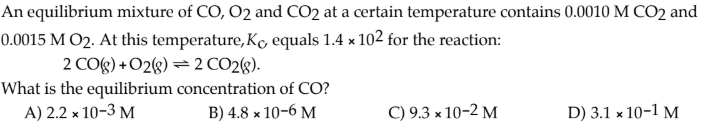# An equilibrium mixture of CO, O2 and CO2 at a certain temperature contains 0.0010 M CO2 and 0.0015 M O2. At this temperature,Kc, equals 1.4 × 10^2 for the reaction: 2 CO(g) +O2(g) ⇌ 2 CO2(g). What is the equilibrium concentration of CO? A) 2.2 × 10^-3 M B) 4.8 × 10^-6 M C) 9.3 × 10^-2 M D) 3.1 × 10^-1 M